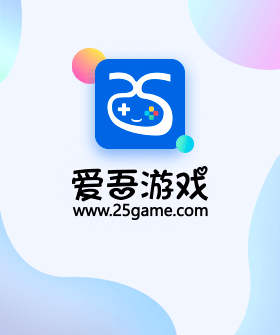# 🎉一飞冲天王者版GM权限表

 GM1商店内容（累计充值10元） 每日限1次，钻石*10=钻石*100000 每日限1次，钻石*10=金币*100000000 每日限1次，钻石*10=进阶石*5000 每日限1次，钻石*10=洗髓丹*1000 每日限1次，钻石*10=经验金车胶囊*1 每日限1次，钻石*10=经验金球胶囊*1 每日限2次，钻石*10=刷新石*10 每日限2次，钻石*10=紫宝物碎片包*1 每日限5次，钻石*10=橙色精灵球胶囊*1 每日限5次，钻石*10=橙色座驾胶囊*1 每日限5次，钻石*10=荣耀套装箱*1 每日限5次，钻石*10=寻宝图*1 每日限5次，钻石*10=氨基酸*20 每日限5次，钻石*10=14级宝石包*1 每日限99次，钻石*10=百变怪*1 每日限99次，钻石*10=白银宝箱*1 每日限99次，钻石*10=白银钥匙*1 GM2商店内容（累计充值30元） 每日限1次，钻石*10=钻石*500000 每日限99次，钻石*10=金币*10000000 每日限1次，钻石*10=经验药*10000 每日限2次，钻石*10=进阶石*5000 每日限2次，钻石*10=洗髓丹*1000 每日限2次，钻石*10=刷新石*20 每日限2次，钻石*10=橙宝物碎片包*1 每日限10次，钻石*10=橙色精灵球胶囊*1 每日限10次，钻石*10=橙色座驾胶囊*1 每日限10次，钻石*10=荣耀套装箱*1 每日限5次，钻石*10=15级宝石包*1 每日限10次，钻石*10=寻宝图*1 每日限5次，钻石*10=刷新石*10 每日限99次，钻石*10=氨基酸*10 每日限99次，钻石*10=黄金宝箱*1 每日限99次，钻石*10=黄金钥匙*1 每日限99次，钻石*10=百变怪*5 每日限99次，钻石*10=紫宝物碎片包*1 GM3商店内容（累计充值100元） 每日限99次，钻石*10=钻石*10000000 每日限99次，钻石*10=经验药*10000 每日限99次，钻石*10=进阶石*5000 每日限10次，钻石*10=洗髓丹*1000 每日限99次，钻石*10=经验药*10000 每日限30次，钻石*10=寻宝图*1 每日限10次，钻石*10=熔炼石*500 每日限10次，钻石*10=15级宝石包*1 每日限10次，钻石*10=海神套装箱*1 每日限99次，钻石*10=橙色精灵球胶囊*1 每日限99次，钻石*10=橙色座驾胶囊*1 每日限99次，钻石*10=荣耀套装箱*1 每日限30次，钻石*10=刷新石*10 GM4商店内容（累计充值200元） 每日限99次，钻石*10=精灵球*1 每日限30次，钻石*10=熔炼石*500 每日限30次，钻石*10=洗髓丹*1000 每日限30次，钻石*10=海神套装箱*1 每日限10次，钻石*10=红色精灵球胶囊*1 每日限10次，钻石*10=红色座驾胶囊*1 每日限30次，钻石*10=15级宝石包*1 每日限99次，钻石*10=橙宝物碎片包*1 每日限10次，钻石*10=淬炼石*500 GM5商店内容（累计充值500元） 每日限99次，钻石*10=海神套装箱*1 每日限30次，钻石*10=淬炼石*500 每日限2次，钻石*10=火箭传说精灵胶囊*1 每日限2次，钻石*10=赤红传说精灵胶囊*1 每日限10次，钻石*10=当世套装箱*1 每日限99次，钻石*10=刷新石*10 每日限99次，钻石*10=寻宝图*1 GM6商店内容（累计充值1000元） 每日限30次，钻石*10=红色精灵球胶囊*1 每日限30次，钻石*10=红色座驾胶囊*1 每日限30次，钻石*10=当世套装箱*1 每日限5次，钻石*10=火箭传说精灵胶囊*1 每日限5次，钻石*10=赤红传说精灵胶囊*1 每日限2次，钻石*10=恶魔之始*10 每日限2次，钻石*10=创世之源*10

## 推荐游戏安卓版下载服务器交互中...